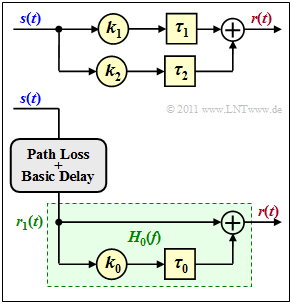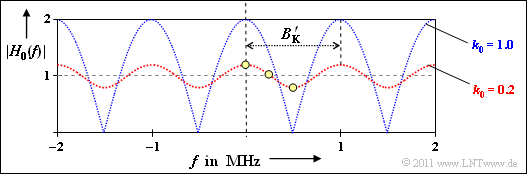# Exercise 2.2: Simple Two-Path Channel ModelTwo equivalent models for the two-path channel

Here we consider a two-path channel for mobile radio according to the adjacent graph, characterized by the model parameters

$$k_1 = 10^{-4}\hspace{0.05cm}, \hspace{0.2cm} \tau_{1} = 10\,{\rm µ s}\hspace{0.05cm}, \hspace{0.2cm}\tau_{2} = 11\,{\rm µ s} \hspace{0.05cm}.$$

Two different numerical values are considered for the damping factor on the secondary path:

• $k_2 = 2 \cdot 10^{-5}$   ⇒   subtasks (1) to (4),
• $k_2 = 10^{-4}$   ⇒   subtasks (5) and (6).

An equivalent channel model is shown below, with only the part highlighted in green being considered further. This means:

• The basic attenuation (path loss) and the basic propagation time are not taken into account here.
• The frequency response of this  $(k_0, \tau_0$)–model is designated  $H_0(f)$.

An important descriptive parameter of any mobile radio system is the coherence bandwidth  $B_{\rm K}$, which is defined in the chapter  The GWSSUS Channel Model . The coherence bandwidth indicates whether the system can be approximated as non–frequency–selective:

• This is justified if the signal bandwidth  $B_{\rm S}$  is significantly smaller than the coherence bandwidth  $B_{\rm K}$.
• Otherwise, the mobile radio system is frequency–selective, which requires a more complicated description.

As a simple approximation for the coherence bandwidth, the reciprocal value of pulse broadening is often used in the literature (marked by an apostrophe in our tutorial):

$$B_{\rm K}\hspace{0.01cm}' = \frac{1}{\tau_{\rm max} - \tau_{\rm min}} \hspace{0.05cm}.$$

Notes:

• This task belongs to the topic of the chapter  Multi–Path Reception in Mobile Communications.
• For the solution you also need the speed of light  $c = 3 \cdot 10^8 \ \rm m/s$.
• For  $k_2$  only positive values are used here. However, as you may remember, if the secondary path is created by reflection on a wall, a phase change by  $\pi$  occurs, resulting in a negative value of  $k_2$.

### Questionnaire

1

What length  $d_1$  does the direct path have?

 $d_1 \ = \$ $\ \ \rm km$

2

What are the parameters of the simplified model for  $k_2 = 2 \cdot 10^{-5}$ ?

 $k_0 \ = \$ $\tau_0 \ = \$ $\ \ \rm µ s$

3

Calculate the magnitude of the frequency response   ⇒   $|H_0(f)|$  of the simplified model for the frequencies  $f = 0$,  $f = 250 \ \rm kHz$  and  $f = 500 \ \rm kHz$ ?

 $|H_0(f = 0)| \ = \$ $|H_0(f = 250 \ \rm kHz)| \ = \$ $|H_0(f = 500 \ \rm kHz)| \ = \$

4

For which signal frequencies  $f_{\rm S}$  does destructive interference occur here?

 $f_{\rm S} = 500 \ \rm kHz$, $f_{\rm S} = 750 \ \rm kHz$, $f_{\rm S} = 1 \ \rm MHz$.

5

What is the approximate coherence bandwidth for  $k_2 = 2 \cdot 10^{-5}$  and  $k_2 = 10^{-4}$ ?

 $k_2 = 2 \cdot 10^{-5} \text{:} \ \hspace{0.4cm} B_{\rm K}\hspace{0.01cm}' = \$ $\ \rm MHz$ $k_2 = 10^{-4} \text{:} \ \hspace{0.4cm} B_{\rm K}\hspace{0.01cm}' = \$ $\ \rm MHz$

6

Which statements are correct regarding frequency selectivity if  $B_{\rm S}$  denotes the signal bandwidth?

 For GSM  $(B_{\rm S} = 200 \ \rm kHz)$:  the channel is frequency selective. For UMTS  $(B_{\rm S} = 5 \ \rm MHz)$:  the channel is frequency selective.

### Solution

#### Solution

(1)  We have  $\tau_1 = d_1/c$  ⇒  $d_1 = \tau_1 \cdot c = 10^{-5} \rm s \cdot 3 \cdot 10^8 \ m/s \ \ \underline {= 3 \ km}$.

(2)  The damping factor is  $k_0 = k_2/k_1 \ \ \underline {= 0.2}$  and the delay time  $\tau_0 = \tau_2 \ - \tau_1 \ \underline {= 1 \ \ \rm µ s}$.

• The effective path loss for both paths is thus  $k_1 = 10^{-4}$  and the basic delay time is  $\tau_1 = 10 \ \ \rm µ s$.

(3)  The impulse location is

$$h_{\rm 0}(\tau) = \delta(\tau) + k_0 \cdot \delta(\tau - \tau_0) \hspace{0.05cm}.$$

By Fourier transformation you get the frequency response

$$H_{\rm 0}(f) \hspace{-0.1cm} \ = \ \hspace{-0.1cm} 1 + k_0 \cdot {\rm e}^{- {\rm j}\cdot 2 \pi f \tau_0}=1 + k_0 \cdot {\cos}( 2 \pi f \tau_0) + {\rm j}\cdot k_0 \cdot {\sin }( 2 \pi f \tau_0) \hspace{0.05cm},$$

and thus to the following magnitude of the frequency response:

$$|H_{\rm 0}(f)| = \sqrt{ \left [ 1 + k_0 \cdot {\cos}( 2 \pi f \tau_0)\right ]^2 + k_0^2 \cdot {\sin^2 }( 2 \pi f \tau_0)}\hspace{0.3cm} \Rightarrow \hspace{0.3cm}|H_{\rm 0}(f = 0)| \hspace{-0.1cm} \ = \ \hspace{-0.1cm} 1+ k_0 \hspace{0.1cm} \underline {=1.2} \hspace{0.05cm},$$
$$|H_{\rm 0}(f = 250\,{\rm kHz})| \hspace{-0.1cm} \ = \ \hspace{-0.1cm} \sqrt{ \left [ 1 + k_0 \cdot {\cos}( \pi/2)\right ]^2 + k_0^2 \cdot {\sin^2 }( \pi/2)} = \sqrt{1+ k_0^2} \hspace{0.1cm} \underline {\approx 1.02} \hspace{0.05cm},$$
$$|H_{\rm 0}(f = 500\,{\rm kHz})| \hspace{-0.1cm} \ = \ \hspace{-0.1cm} \sqrt{ \left [ 1 + k_0 \cdot {\cos}( \pi)\right ]^2 + k_0^2 \cdot {\sin^2 }( \pi)} = {1- k_0} \hspace{0.1cm} \underline {= 0.8} \hspace{0.05cm}.$$Magnitude of the frequency response of a two-way channel

The graphic (red curve) shows the function  $|H_0(f)|$.

• The values you are looking for are marked by the yellow dots.
• The blue curve refers to  (5)  with  $k_0 = 1 \ \Rightarrow \ k_2 = k_0 \cdot k_1 = 10^{-4}$.

(4)  Solution 1 is correct:

• Destructive interference occurs for $|H_0(f)| < 1$, e.g. for $f = 500 \ \rm kHz$.
• On the other hand:
$$|H_{\rm 0}(f = 750\,{\rm kHz})| \hspace{-0.1cm} \ = \ \hspace{-0.1cm} |H_{\rm 0}(f = 250\,{\rm kHz})| \approx 1.02 > 1\hspace{0.05cm},$$
$$|H_{\rm 0}(f = 1\,{\rm MHz})| \hspace{-0.1cm} \ = \ \hspace{-0.1cm} |H_{\rm 0}(f = 0)| = 1.2 > 1 \hspace{0.05cm}.$$

(5)  The difference  $\tau_{\rm max} - \tau_{\rm min}$  between the delays in the two paths is equal to  $\tau_0 = 1 \ \ \rm µ s$.

• So the coherence bandwidth is
$$B_{\rm K}\hspace{0.01cm}' = {1}/{\tau_{\rm 0} } \hspace{0.1cm} \underline {=1\,{\rm MHz}} \hspace{0.05cm}.$$
• The result is independent from  $k_2$.  It applies to  $k_2 = 2 \cdot 10^{-5} \Rightarrow \ k_0 = 0.2$  and  $k_2 = 10^{-4} \Rightarrow \ k_0 = 1$  in the same way.
• This approximation  $B_{\rm K}\hspace{0.01cm}'$  of the coherence bandwidth is shown in the graph.

(6)  Solution 2 is correct:

• The channel is non–frequency–selective if the coherence bandwidth  $B_{\rm K}$  is significantly larger than the signal bandwidth  $B_{\rm S}$.
• For the given channel, this is true for GSM, but not for UMTS. For UMTS, this is a frequency selective channel.